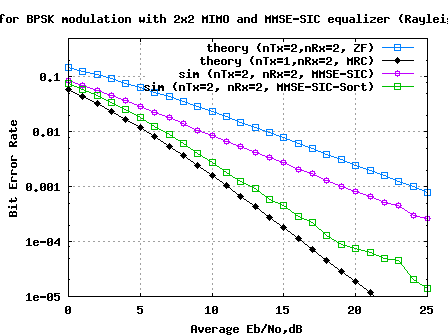- DSP log - http://www.dsplog.com -

MIMO with MMSE SIC and optimal ordering

Posted By Krishna Sankar On December 6, 2008 @ 2:56 pm In Channel,MIMO | 26 Comments

This post attempts to build further on the MIMO equalization schemes which we have discussed -

(a) Minimum Mean Square Error (MMSE)  equalization,

(c) ZF-SIC with optimal ordering .

We have learned that successive interference cancellation with optimal ordering improves the performance with Zero Forcing equalization. In this post, we extend the concept of successive interference cancellation to the MMSE equalization and simulate the performance. We will assume that the channel is a flat fading Rayleigh multipath channel  and the modulation is BPSK.

Brief description of 2×2 MIMO transmission, assumptions on channel model and the noise are detailed in the post on Minimum Mean Square Error (MMSE)  equalization.

## MMSE equalizer for 2×2 MIMO channel

Let us now try to understand the math for extracting the two symbols which interfered with each other. In the first time slot, the received signal on the first receive antenna is,

.

.

where

, are the received symbol on the first and second antenna respectively,

is the channel from transmit antenna to receive antenna,

is the channel from transmit antenna to receive antenna,

is the channel from transmit antenna to receive antenna,

is the channel from transmit antenna to receive antenna,

, are the transmitted symbols and

is the noise on receive antennas.

We assume that the receiver knows , , and . The receiver also knows and . For convenience, the above equation can be represented in matrix notation as follows:

.

Equivalently,

The Minimum Mean Square Error (MMSE) approach tries to find a coefficient which minimizes the criterion,

.

Solving,

.

Using the Minimum Mean Square Error (MMSE) equalization, the receiver can obtain an estimate of the two transmitted symbols , , i.e.

.

## Successive Interference Cancellation

#### (a) Simple

In classical Successive Interference Cancellation, the receiver arbitrarily takes one of the estimated symbols (for example the symbol transmitted in the second spatial dimension, ), and subtract its effect from the received symbol and . Once the effect of is removed, the new channel becomes a one transmit antenna, 2 receive antenna case and can be optimaly equalized by Maximal Ratio Combining  (MRC).

#### (b) With optimal ordering

However, we can have more intelligence in choosing whether we should subtract the effect of first or first. To make that decision, let us find out the transmit symbol (after multiplication with the channel) which came at higher power at the receiver. The received power at the both the antennas corresponding to the transmitted symbol is,

.

The received power at the both the antennas corresponding to the transmitted symbol is,

.

If then the receiver decides to remove the effect of from the received vector and . Else if the receiver decides to subtract effect of from the received vector and , and then re-estimate .

Once the effect of either or is removed, the new channel becomes a one transmit antenna, 2 receive antenna case and the symbol on the other spatial dimension can be optimally equalized by Maximal Ratio Combining  (MRC).

For detailed equations on the contruction of the new 2 x 1 channel using successive interference cancellation, please refer to the post on ZF-SIC with optimal ordering. 

## Simulation Model

The Matlab/Octave script performs the following

(a) Generate random binary sequence of +1′s and -1′s.

(b) Group them into pair of two symbols and send two symbols in one time slot

(c) Multiply the symbols with the channel and then add white Gaussian noise.

(d) Equalize the received symbols with Minimum Mean Square Error criterion

(e) Do successive interference cancellation by both classical and optimal ordering approach

(f) Perform Maximal Ratio Combining for equalizing the new received symbol

(g) Perform hard decision decoding and count the bit errors

(h) Repeat for multiple values of and plot the simulation and theoretical results.Figure; BER plot for 2×2 MIMO channel with MMSE-SIC equalization with and without optimal ordering

## Observations

Compared to Minimum Mean Square Equalization with simple successive interference cancellation case, addition of optimal ordering results in around 5.0dB of improvement for BER of .The performance is now closely matching with curve 1 transmit 2 receive antenna MRC case.

## References

[DIG-COMM-BARRY-LEE-MESSERSCHMITT] Digital Communication: Third Edition, by John R. Barry, Edward A. Lee, David G. MesserschmittURL to article: http://www.dsplog.com/2008/12/06/mimo-with-mmse-sic-and-optimal-ordering/

URLs in this post:

 Minimum Mean Square Error (MMSE): http://www.dsplog.com/2008/11/02/mimo-mmse-equalizer/

 Zero Forcing equalization with Successive Interference Cancellation (ZF-SIC): http://www.dsplog.com/2008/11/09/mimo-zero-forcing-successive-interference-cancellation/

 ZF-SIC with optimal ordering: http://www.dsplog.com/2008/11/29/mimo-zf-sic-optimal-order/

 Rayleigh multipath channel: http://www.dsplog.com/2008/07/14/rayleigh-multipath-channel/

 Maximal Ratio Combining: http://www.dsplog.com/2008/09/28/maximal-ratio-combining/

 Matlab/Octave script for simulating BER for BPSK in 2×2 Rayleigh fading MIMO channel with MMSE-SIC equalization with and without optimal ordering: http://www.dsplog.com/db-install/wp-content/uploads/2008/12/script_ber_mimo_mmse_sic_bpsk_rayleigh_channel.m